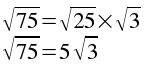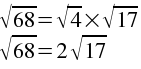SEARCH HOMEMath Central Quandaries & QueriesQuestion from Melissa, a student: How do you simplify the square root of 164?Hi Melissa.

When you "simplify" a square root, you simply factor out all the perfect squares that are factors.

For example, to simplify the square root of 75, first I recognize that the perfect square 25 goes into it.

So 75 = 25 x 3. Therefore the square root of 75 equals the square root of 25 times the square root of 3. And that means just 5 times the square root of 3.Here's another example: Simplify the square root of 68. Looking at it, I notice that 4 (a perfect square) goes into that. 68 = 4 x 17. And since 4 = 2^2, then 68 simplifies to 2 times the square root of 17.Now you try simplifying the square root of 164. What perfect squares go into 164?

Hope this helps,
Stephen La RocqueMath Central is supported by the University of Regina and The Pacific Institute for the Mathematical Sciences.